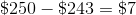HSPT Math : Problem Solving

Example Questions

Example Question #6 : Percentages

To get on the ballot for student body president, a student must turn in a petition with the signatures of 3% of the students. If there are 5,319 students, how many signatures must a student get to be on that ballot? (Nearest whole person)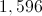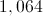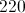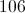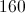Explanation:

3% of 5,319 can be calculated by multiplying 5,319 by 0.03, the decimal equivalent of 3%: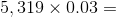Multiply 5,319 by 3, then move the decimal point so that two digits are to the right: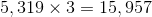,

so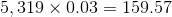Rounded to the nearest whole number, this is 160 signatures.

A laptop computer is on sale for 40% less than the original price. If the original price is $345.00, what is the laptop's current sale price? Possible Answers: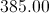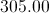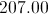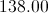Correct answer:Explanation: First, multiply the original price by the sale percentage: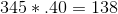Then, subtract that amount from the original price to get the sale price: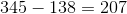. The laptop's current sale price is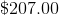Example Question #8 : Percentages Helen wants to buy a dress for a party. It is on sale for 37% off. How much does the dress currently costs, if its original price was$45.00?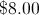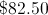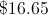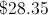Explanation:

First, multiply 45 by 0.37: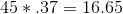Then, subtract that amount from 45.00 to find the sale price: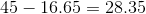Answer: The dress' sale price is $28.35. Example Question #1 : How To Find The Part From The Whole With Percentage A restaurant is having a sale and lowering their prices by 20%. If an average meal for two normally costs$62.00, what will it costs under the current sale?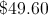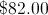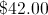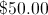Explanation:

Multiply: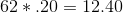Then, subtract that amount from the original price: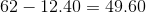Answer: $49.60 Example Question #2 : How To Find The Part From The Whole With Percentage Candy bought a sweater and a beanie on sale for 20% off. If the sweater cost$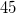and the beanie cost $, how much did Candy spend? Possible Answers:$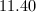$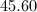$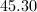$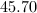$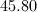$Explanation: In order to find how much money Candy spent, we must first figure out the cost of the merchandise before the discount. We do this by adding the prices together.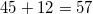Next we must find 20% of$57.00.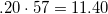Since 20% is the percentage Candy saved, we must subtract $11.40 from the original price.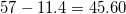So Candy spent$45.60.

Donald makes $17.50 an hour at current, and is due for a 5% raise. Also, as a result of an agreement with the union, management has promised a 16% increase in wages across the board for all workers. What will Donald earn per hour after these two increases? Possible Answers: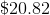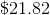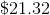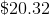Correct answer:Explanation: As a result of a 5% raise, Donald's wages will be 105% of what they were before; the 16% wage hike will make Donald's wages 116% of that. Therefore, Donald will make: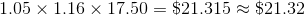Example Question #71 : Problem Solving In appreciation for a job well done, Clark is being awarded a bonus equal to 12.5% of the salary he earned over the last two years. His monthly salary over that period has remained constant at$4,500 per month. What is the amount of the bonus?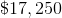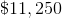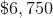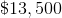Explanation:

Over two years, or 24 months, Clark has earned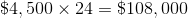His bonus is 12.5% of this, or: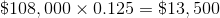Example Question #13 : Percentages

Jerry buys groceries that cost $43.23 before tax. The tax on groceries in his locality is 5.5%. He hands the cashier a$100 bill. What will he get back in change?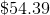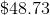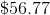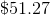Explanation:

The tax on $43.23 worth of groceries is 5.5% of that, which is 0.055 multiplied by that: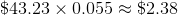when rounded to the nearest cent. Add that to the price: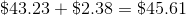And subtract that from$100: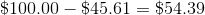, which is the change

Example Question #14 : Percentages

At the store where Harry works, a hat he wants to buy sells for $72.00. He gets an employee discount of 20%, and he wants to hold out for two weeks, when the hat is marked down 15%. How much will he be able to buy the hat for then? Possible Answers: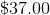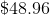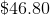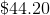Correct answer:Explanation: A 20% employee discount means that Harry gets to buy merchandise for 80% of the purchase price. A 15% markdown means that the hat will sell for 85% of its normal price. Therefore, Harry will pay 80% of 85% of$72 for the hat, or: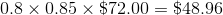Example Question #15 : Percentages

A bicycle Carly wants to buy costs $250 in her hometown in Tennessee, where she would pay no sales tax. Just across the border in Kentucky, she can buy the same bicycle for$225 in a store, but the sales tax is 8%. How much would Carly save by buying the bicycle in Kentucky?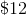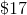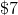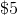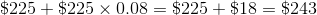She would save